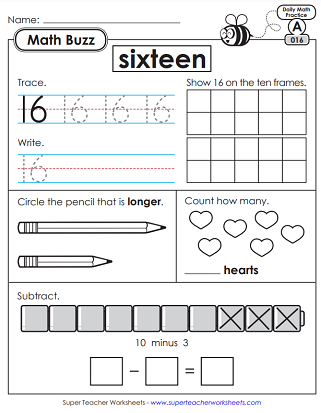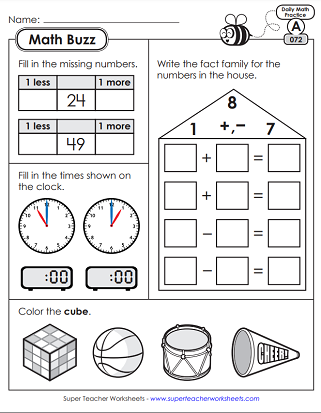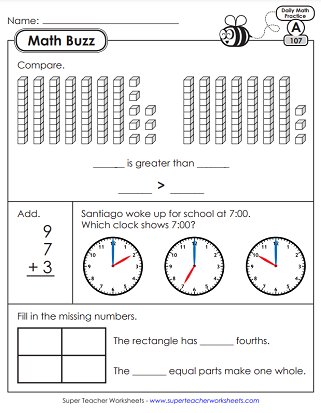# Daily Math Review Worksheets - Level A (1st Grade)

Math Buzz is a set of 150 daily, spiraled review worksheets for 1st graders. Each file contains five worksheets, one for each day of the week. Students begin by reviewing basic Kindergarten concepts. The series then evolves to reinforce more advanced 1st grade skills.In the first week of 1st grade Math Buzz, students will review basic Kindergarten skills. This file has 5 worksheets, one for each day of the week. Skills include basic addition, counting on a five-frame, writing numbers, and identifying basic shapes.
Skills include counting, tracing, and writing numbers to ten. Students also add numbers with sums up to 10 using visual models. There are also questions related to more/less and longer/shorter.
Count, trace, and write numbers 11-15. Subtract numbers using illustrations. Identify basic shapes. Compare single-digit numbers.
Trace numbers. Show numbers on a ten-frame. Add and subtract with graphical models of manipulatives. Classify shapes. Identify correct preposition words.
Solve to find missing addends in an equation. Count corners on a polygon. Find and identify rectangles and circles. Solve simple addition and subtraction word problems.
This week, students practicing counting and writing numbers up to 30. Calculate missing addends in addition equations. Identify longer/shorter and long/longer/longest objects.
Your students will review place value, identify the number of tens and ones in a 2-digit number. Add zero to single-digit numbers. Identify a polygons, including hexagons. Count up from 2-digit numbers.
Review basic addition and subtraction facts. Practice breaking a 2-digit number up into tens and ones. Compare numbers, using the phrases less than and greater than. Answer a question using a pictograph.
Identify solid shapes (spheres, cubes, rectangular prisms). Answer questions from a pictograph. Complete a bar model. Fill in the missing parts on a tally chart.
For the tenth week of M.B., students will order objects by length (shortest to longest), identify solid shapes (cubes, cones, spheres), complete a pictograph, and answer place value questions.
This week you students will decompose 2-digit numbers into 1s and 10s, draw a model to solve an addition problem, determine the number of straight sides on polygons, and continue shapes in a pattern.
Draw a model to solve an addition problem. Answer a question with tally marks. Show "one less" than the given numbers. Solve basic subtraction problems.
In this set, pupils will identify cubes, spheres, and cylinders. They will also complete an addition bar model, use non-standard units to measure a lizard, and compare two digit numbers (using <, >, and =).
On these Math Buzz worksheets, your first graders will measure a whale using non-standard units, identify solids (cones, cylinders, cubes), subtract, and identify shapes that have equal parts.
In this week's worksheet set, students will practice counting by 10s (6, 16, 26, 36...), find "one more" and "one less" than a given number, write the times shown on the analog clocks (11:00 and 1:00), and illustrate a math fact family.
On these worksheets, students will count by tens starting with (8, 18, 28, 38...), complete a part-part-whole diagram, complete a fact family, and use place value blocks to find a number.
Find the missing numbers (by adding and subtracting one). Determine which 3D shaped have curved surfaces. Answer questions about a pictograph. Tell how many equal parts are shown on circles. Measure a shoe using non-standard units.
Tell the time shown on the analog clocks. Tell how many equal parts are in each fraction diagram. Measure a flashlight using nonstandard units. Compare 2-digit numbers.
Complete a number bond (fact family). Tell how many tens and ones it takes to make 43. Tell time on an analog clock. Measure a glue stick using non-standard units.
Color the date on a calendar. Measure a marker with non-standard units. Compare two digit numbers using <, >, and =. Solve basic addition and subtraction word problems.
Draw hands on a clock. Find related math facts. Add using place value blocks. Solve addition/subtraction word problems. Find the sum of three single-digit addends.
Add and using place value blocks. Solve addition and subtraction word problems. Draw hands on an analog clock (nearest hour). Identify flat faces of a cylinder.
Find 10 more and 10 less than a given number. Solve a column addition problem (2-digit plus 1-digit). Identify flat surfaces of a cone. Measure a pencil and crayon with non-standard units.
Use non-standard units to compare lengths of a guitar and a drum. Use place value blocks to solve subtraction and addition problems. Answer questions about a bar graph. Draw hands on a clock.
Review fact families, telling time on analog clocks, 3D shapes, and US coin identification.
Practice addition problems with missing addends, comparing sizes (shorter, longer), drawing lines to divide a shape into quarters, add and subtract double-digit numbers, and make a pictograph based on data in a tally table.
Demonstrate knowledge of place value (tens and ones), coin identification (penny), comparing measurements in non-standard units, identifying halves, and solving word problems.
This set of Math Buzz worksheets has more place value questions, coin identification (dime and quarter), some 10 more/10 less problems, and simple addition with three addends.
This week, students will find the total value of the coins shown (pennies and nickels), compare sums (using <, >, or =), solve word problems, and tell time on an alaong clock to the nearest half hour.
In the final first grade Math Buzz worksheet set, students will draw coins to make 7 cents, find sums and compare, draw lines to divide circles into halves and quarters, find sums and differences.
More Daily Review (Math Buzz)

Super Teacher Worksheets has Math Buzz worksheets for all grade levels. If your students need more of a challenge, consider jumping ahead to the 2nd grade series.

## Sample Worksheet ImagesMy Account
Site Information